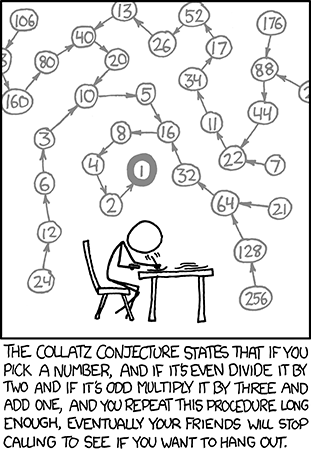# C++: Hailstone Sequence

Posted in C++The Hailstone sequence of numbers can be generated from a starting positive integer, n by:

• If n is 1 then the sequence ends.
• If n is even then the next n of the sequence `= n/2`
• If n is odd then the next n of the sequence `= (3 * n) + 1`

The (unproven), Collatz conjecture is that the hailstone sequence for any starting number always terminates.

1. Create a routine to generate the hailstone sequence for a number.
2. Use the routine to show that the hailstone sequence for the number 27 has 112 elements starting with `27, 82, 41, 124` and ending with `8, 4, 2, 1`
3. Show the number less than 100,000 which has the longest hailstone sequence together with that sequence’s length.
(But don’t show the actual sequence!)

```#include <iostream>
#include <vector>
#include <utility>

std::vector<int> hailstone(int i)
{
std::vector<int> v;
while(true){
v.push_back(i);
if (1 == i) break;
i = (i % 2) ? (3 * i + 1) : (i / 2);
}
return v;
}

std::pair<int,int> find_longest_hailstone_seq(int n)
{
std::pair<int, int> maxseq(0, 0);
int l;
for(int i = 1; i < n; ++i){
l = hailstone(i).size();
if (l > maxseq.second) maxseq = std::make_pair(i, l);
}
return maxseq;
}

int main () {

// Use the routine to show that the hailstone sequence for the number 27
std::vector<int> h27;
h27 = hailstone(27);
// has 112 elements
int l = h27.size();
std::cout << "length of hailstone(27) is " << l;
// starting with 27, 82, 41, 124 and
std::cout << " first four elements of hailstone(27) are ";
std::cout << h27 << " " << h27 << " "
<< h27 << " " << h27 << std::endl;
// ending with 8, 4, 2, 1
std::cout << " last four elements of hailstone(27) are "
<< h27[l-4] << " " << h27[l-3] << " "
<< h27[l-2] << " " << h27[l-1] << std::endl;

std::pair<int,int> m = find_longest_hailstone_seq(100000);

std::cout << "the longest hailstone sequence under 100,000 is " << m.first
<< " with " << m.second << " elements." <<std::endl;

return 0;
}
```
Output:
``` length of hailstone(27) is 112 first four elements of hailstone(27) are 27 82 41 124
last four elements of hailstone(27) are 8 4 2 1
the longest hailstone sequence under 100,000 is 77031 with 351 elements.```

SOURCE

Content is available under GNU Free Documentation License 1.2.